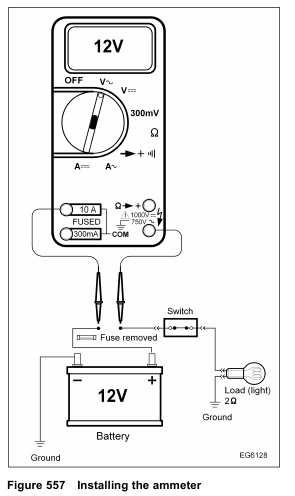# International DT466 – DIAGNOSTIC TOOLS AND ACCESSORIES – Electronic Circuit Testing – Ammeter

An ammeter measures current flow (amperage) in a circuit. Amperes (or amps) are units of electron flow
that indicate how many electrons are passing through the circuit. An amp is the unit of measurement for the
current flow in the circuit.
Ohm’s Law states that the current flow is equal to the circuit voltage divided by the total circuit resistance (I = E ÷ R). Therefore, increasing the voltage also increases the current flow. Any decrease in resistance will also increase the current flow.

At normal operating voltage, most circuits have a characteristic amount of current flow (current draw).
Current draw can be measured with an ammeter. Valuable diagnostic information can be provided by referring to a specified current draw rating for a component (electrical device), measuring the current flow in the circuit, and then comparing the two measurements (the specified current draw versus the actual measurement).An ammeter is connected in series with the load, switches, resistors, etc., so that all of the current flows through the meter. The ammeter measures current flow only when the circuit is powered up and operating. The DMM is fused to measure up to 10 amps using the 10 A connection point.
Before measuring current flow, determine approximately how many amps are in the circuit to correctly connect the ammeter. The estimate of current flow can easily be calculated. The resistance of the light bulb is 2 ohms. Applying Ohm’s law, current flow will be 6 amps (6 amps = 12 V ÷ 2 ohms). If the fuse is removed and an ammeter is installed with the switch closed, 6 amps of current will be measured flowing in the circuit. Notice that the ammeter is installed in series so that all the current in the circuit flows through it.

Excessive current draw means that more current is flowing in a circuit than the fuse and circuit were designed to handle. Excessive current draw will open fuses and circuit breakers, and will also quickly discharge batteries. An ammeter can diagnose these conditions.
Reduced current draw will cause a device (an electric window motor, for example) to operate poorly. Increased circuit resistance will cause lower current flow (often due to loose or corroded connections).